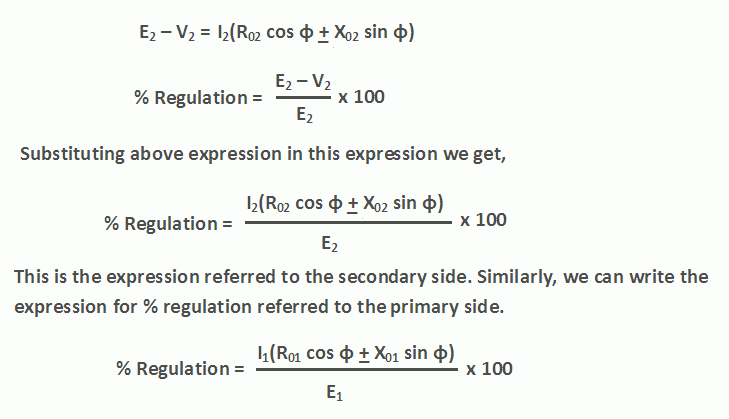# Voltage Regulation of Transformer

The voltage regulation of transformer is defined as the change in secondary terminal voltage (V2) from no-load to full load at constant primary voltage and temperature. It is expressed as a percentage of the secondary no-load voltage.

Mathematically, % Regulation of transformer = (E2 – V2) x 100 / E2

No-load voltage: The secondary terminal voltage of transformer when no load is connected to the transformer is known as the no-load voltage of the transformer. At no load, the secondary terminal voltage will be equal to induced EMF in the secondary winding.

So, no-load voltage = E2 volts

Full load voltage: It is the secondary terminal voltage of transformer when a rated load is connected to the transformer. We will denote it by V2.

When a transformer is loaded a voltage drop in primary and secondary impedances of transformer takes place. As the load current increases, this voltage drop will increase. This will reduce the secondary terminal voltage V2. The ideal value of voltage regulation of transformer is 0%.

# Voltage Regulation of Transformer | Formula

The approximate expression for the total voltage drop (E2 – V2) in a transformer as referred to secondary is given byWhere,
R02 = equivalent resistance of transformer referred to secondary
X02 = equivalent reactance of transformer referred to secondary
R01 = equivalent resistance of transformer referred to primary
X01 = equivalent resistance of transformer referred to primary

Here it has been assumed that φ1 = φ2 = φ.

The positive sign is used for a lagging power factor and the negative sign for a leading power factor.

It is clear from the above expressions, the voltage regulation of transformer does not depend only on the magnitude of load current. But it also depends on the type of load. The transformer regulation is positive for the resistive and inductive loads but it can be negative for the capacitive loads.

We can determine the values of R01, X01, R02, X02 of a transformer from short circuit test and calculate percentage regulation of transformer.

## Calculate Voltage Regulation of Transformer

Example: A 100 kVA transformer has 400 turns on the primary and 80 turns on the secondary. The primary and secondary resistances are 0.3 Ω and 0.01 Ω respectively and the corresponding leakage reactances are 1.1 and 0.035 Ω respectively. The supply voltage is 2200 V. Calculate:

• equivalent impedance referred to primary and,
• the voltage regulation and the secondary terminal voltage for full load having a power factor of 0.8 leading.

Solution: K = 80/400 = 1/5,

R1 = 0.3 Ω,
R01 = R1 + R2/K2 = 0.3 + 0.01/(1/5)2 = 0.55 Ω
X01 = X1 + X2/K2 = 1.1 + 0.035/(1/5)2 = 1.975 Ω
Z01 = 0.55 + j 1.975 = 2.05 ∠74.44o

Z02 = K2Z01 = (1/5)2 (0.55 + j 1.975) = (0.022 + j 0.079)

No-load secondary voltage = KV1 = (1/5) × 2200 = 440 V,
I2 = 10 × 103/440 = 227.3 A

Full-load voltage drop as referred to secondary
= I2 (R02 cos φ − X02 sin φ)

= 227.3 (0.022 × 0.8 − 0.079 × 0.6 ) = − 6.77 V

% regn. = − 6.77 × 100/440 = − 1.54

Secondary terminal voltage on load = 440 − (− 6.77) = 446.77 V

## Transformer | All Posts

1.